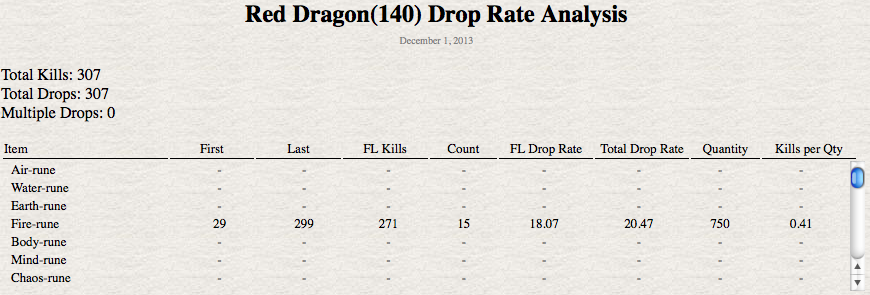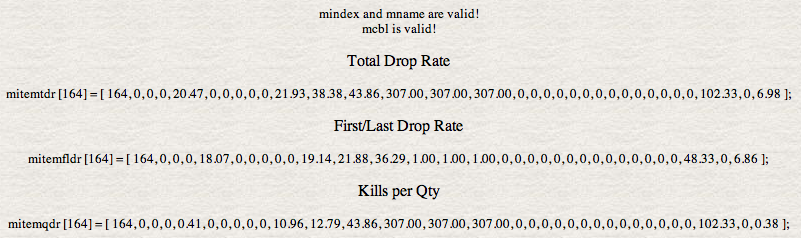December 6, 2013

These pages analyze the rate of selected dropped items. This data is used to build the data base used by the special guide, The Best Droppers in RuneScape Classic.An analysis page is divided into 2 sections. The top section contains the title, general information and drop rate analysis table. The drop rate of a particular item is calculate 3 ways. The drop rate indicates how many kills on average it will take to acquire an item. The lower the drop rate is the fewer kills required to receive the drop of a particular item.

Note: All rates are rounded to 2 decimal places in the analysis pages. The Drop Tables round to 1 decimal place.

First to Last Drop Rate:

The kill count from the first kill that produces a drop of an item to the last kill that produces a drop of that item is determined. The First/Last Drop Rate is computed by dividing the kill count by the number of times a kill produced a drop of the item being examined.

In the example above:

The First (kill) is 29 and the last (kill) is 299. The kill count is 299 - 29 + 1 (count the first kill) = 271.

The number of drops found is shown in the Count column which is 15.

The FL Drop Rate = 271 / 15 = 18.07. On average you should get a Fire-rune drop every 18 kills.

Note: The FL Drop Rate is not used or shown in the Drop Tables. Also, First to Last requires at least 2 drops to have a useable drop rate value.

Total Drop Rate:

The Total Drop Rate is computed by dividing the Total Kills by the number of times a kill produced a drop of the item being examined.

In the example above:

The Total Kills is 307.

The number of drops found is shown in the Count column which is 15.

The Total Drop Rate = 307 / 15 = 20.47. On average you should get a Fire-rune drop every 21 kills.

Note: The Total Drop Rate is what is shown in the Drop Tables.

Kills per Qty:

The Kills per Qty Drop Rate is computed by dividing the Total Kills by the quantity of an item produced by all the kills that produce the item type being examined.

In the example above:

The Total Kills is 307.

The quantity dropped is shown in the Quantity column which is 750.

The Total Drop Rate = 307 / 750 = 0.41. On average you should get around 2 Fire-runes every kill.

Note: The Kills per Qty Drop Rate can appear in the Drop Tables.

So which to use?

That depends on what you are looking for.

The FL Drop Rate is generally more accurate for the Common and UnCommon drops.

The Total Drop Rate is the most accurate way to determine a drop rate for any item when the total drops exceed twice the extremely rare drop rate.

The Kills per Qty Drop Rate is used when you are looking to maximize the quantity of a particular item, as with runes. A monster who drops an item at a low drop rate but in high quantity can produce more items in a long period of time relative to a monster who drops the item often but in small quantities.The bottom section helps me create the data bases used by special guide, The Best Droppers in RuneScape Classic. There are 3 data bases used for each monster. Each data base holds the information for a particular type of drop rate.